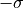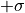# Normalize Image#

## Synopsis#

Normalize an image.

## Code#

### C++#

#include "itkImage.h"
#include "itkNormalizeImageFilter.h"
#include "itkStatisticsImageFilter.h"

#ifdef ENABLE_QUICKVIEW
#  include "QuickView.h"
#endif

#include <iomanip>

int
main(int argc, char * argv[])
{
if (argc < 2)
{
std::cerr << "Usage: " << argv << " filename" << std::endl;
return EXIT_FAILURE;
}

using FloatImageType = itk::Image<double, 2>;

using NormalizeFilterType = itk::NormalizeImageFilter<FloatImageType, FloatImageType>;
auto normalizeFilter = NormalizeFilterType::New();
normalizeFilter->SetInput(input);

using StatisticsFilterType = itk::StatisticsImageFilter<FloatImageType>;
auto statistics1 = StatisticsFilterType::New();
statistics1->SetInput(input);

auto statistics2 = StatisticsFilterType::New();
statistics2->SetInput(normalizeFilter->GetOutput());

#ifdef ENABLE_QUICKVIEW
QuickView viewer;

std::stringstream desc1;
statistics1->Update();
desc1 << itksys::SystemTools::GetFilenameName(argv) << "\nMean: " << statistics1->GetMean()
<< " Variance: " << statistics1->GetVariance();

std::stringstream desc2;
statistics2->Update();
desc2 << "Normalize"
<< "\nMean: " << std::fixed << std::setprecision(2) << statistics2->GetMean()
<< " Variance: " << statistics2->GetVariance();

viewer.Visualize();
#endif
return EXIT_SUCCESS;
}


## Classes demonstrated#

template<typename TInputImage, typename TOutputImage>
class NormalizeImageFilter : public itk::ImageToImageFilter<TInputImage, TOutputImage>

Normalize an image by setting its mean to zero and variance to one.

NormalizeImageFilter shifts and scales an image so that the pixels in the image have a zero mean and unit variance. This filter uses StatisticsImageFilter to compute the mean and variance of the input and then applies ShiftScaleImageFilter to shift and scale the pixels.

NB: since this filter normalizes the data such that the mean is at 0, andtois mapped to -1.0 to 1.0, output image integral types will produce an image that DOES NOT HAVE a unit variance due to 68% of the intensity values being mapped to the real number range of -1.0 to 1.0 and then cast to the output integral value.

See

NormalizeToConstantImageFilter

ITK Sphinx Examples: